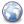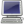# 最大流-Dinic算法和SAP算法简介

Dinic 算法的基本步骤为:

1) 计算残余网络的层次图。我们定义 h[i] 为顶点 i 距离源 S 所经过到最小边数，求出所有顶点的 h 值，h[] 值相同的顶点属于同一层，这就是网络的层次图。

2) 在层次图上进行 BFS 增广，直到不存在增广路径。这时求得的增广路径上顶点是分层的，路径上不可能存在两个顶点属于同一层，即 h[i]== h[j] (i!= j )。同时，求得层次图后，我们可以在层次图上进行多次增广。

3) 重复 1 和
2。直到不存在增广路径。

/*
Pku 3469 Dual Core CPU ( Dinic )
*/
#include <stdio.h>
#include <stdlib.h>
#include <string.h>

int const N= 20010, M= 880010;
int n, m, idx= -1, S, T;

#define min(a,b) ( (a)< (b)? (a): (b) )

struct Edge{
int wt, v;

Edge* next;
}tb[M];

Edge* mat[N];
int h[N], que[N];

void inline add( int u, int v, int x, int y ){

idx++;

tb[idx].wt= x; tb[idx].v= v;

tb[idx].next= mat[u]; mat[u]= tb+ idx;

idx++;

tb[idx].wt= y; tb[idx].v= u;

tb[idx].next= mat[v]; mat[v]= tb+ idx;
}

inline Edge* reserve( Edge* p ){

return tb+ ((p-tb)^1);
}

int bfs(){

int head= 0, tail= 0;

for( int i= 0; i<= T; ++i ) h[i]= -1;

que= S; h[S]= 0;

while( head<= tail ){

for( Edge* p= mat[u]; p; p= p->next ){

int v= p->v, w= p->wt;

if( h[v]== -1 && w> 0 ){

h[v]= h[u]+ 1; que[++tail]= v;

}

}

}

return h[T]!= -1;
}

int dfs( int u, int flow ){

if( u== T ) return flow;

int tf= 0, f;

for( Edge* p= mat[u]; p; p= p->next ){

int v= p->v, w= p->wt;

if( h[v]== h[u]+ 1 && w> 0 && tf< flow && ( f= dfs( v, min( w, flow- tf ) ) ) ){

p->wt-= f;

reserve(p)->wt+= f;

tf+= f;

}

}

if( tf== 0 ) h[u]= -1;

return tf;
}

char ch;

int d;

while( ch= getchar(), ch== ' ' || ch== 'n');

d= ch- '0';

while( ch= getchar(), ch>= '0' && ch<= '9' )

d= d* 10+ ch- '0';

return d;
}

int main(){

scanf("%d%d",&n,&m );

S= 0, T= n+ 1; idx= -1;

for( int i= 0; i<= n+ 1; ++i ) mat[i]= 0;

for( int i= 1; i<= n; ++i ){

int x, y;

add( S, i, x, 0 ); add( i, T, y, 0 );

}

for( int i= 0; i< m; ++i ){

int x, y, z;

add( x, y, z, z );

}

int ans= 0;

while( bfs()) ans+= dfs( S, 0x7fffffff );

printf("%dn", ans );

return 0;
}

SAP 计算的是反向图的层次图，这和原图的层次图是作用是一样的，当然其实Dinic也可以计算反向图的层次图。确保增广路径最短，SAP 意思就是 Shortest Augmenting Paths，最短增广路径。计算反向图的层次图是便于重新给顶点标号，即重新确定其层次图。具体做法为，当我们找到一条经过顶点 i 的增广路径后，对于所有边<i,j>，计算出 m= min{ h[j] ]，这是我们就可以把 i 重新标号为 h[i]= min+ 1。实际上，我们可以首先不需要计算反向图的层次图，而是把所有顶点的层次标为0，这对效率没多大影响。然则这样优化后还不够，又出现了一个 gap 优化。所谓 gap 优化就是计算出层次图后，层次出现断层，这是可以确定残余网络中不存在增广路径了，算法就可以提前结束。这个优化看似微小，实际作用确不小。做法就是保存某一个标号在残余网络中出现的次数，如果是 0 ，就断层了。

### 3 则回应给 最大流-Dinic算法和SAP算法简介

1.huazheng说：Well,I’m no good at making speeches,…so…have a wonderful time doing what u like…and,see u in Spring Festival.

•rexdf说：e.. When starting do a website… we all hope more people to visit

•rexdf说：现在回复应该有邮件了